×#### Thank you for registering.

One of our academic counsellors will contact you within 1 working day.

Click to Chat

1800-1023-196

+91-120-4616500

CART 0

• 0

MY CART (5)

Use Coupon: CART20 and get 20% off on all online Study Material

ITEM
DETAILS
MRP
DISCOUNT
FINAL PRICE
Total Price: Rs.

There are no items in this cart.
Continue Shopping• Complete JEE Main/Advanced Course and Test Series
• OFFERED PRICE: Rs. 15,900
• View Details

```Chapter 8: Lines And Angles Exercise – 8.4

Question: 1

In below fig. AB CD and ∠1 and ∠2 are in the ratio 3: 2. Determine all angles from 1 to 8.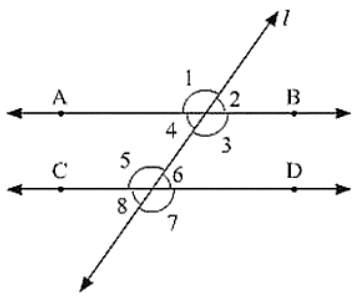Solution:

Let ∠1 = 3x and ∠2 = 2x

∠1 and ∠2 are linear pair of angle

Now, ∠1 and ∠2

⟹ 3x + 2x = 180

⟹ 5x = 180

⟹ x = 180/5

⟹ x = 36

∠1 = 3x = 108°,∠2 = 2x = 72°

We know, Vertically opposite angles are equal

∠1 = ∠3 = 108°

∠2 = ∠4 = 72°

∠6 = ∠7 = 108°

∠5 = ∠8 = 72°

We also know, corresponding angles are equal

∠1 = ∠5 = 108°

∠2 = ∠6 = 72°

Question: 2

In the below fig, I, m and n are parallel lines intersected by transversal p at X. Y and Z respectively. Find ∠1, ∠2 and ∠3Solution:

From the given figure:

∠3 + ∠mYZ = 180°       [Linear pair]

⟹ ∠3 = 180 − 120

⟹ ∠3 = 60°

Now line I parallel to m

∠1 = ∠3             [Corresponding angles]

∠1 = 60°

Also m parallel to n

⟹ ∠2 = 120°          [Alternative interior angle]

Hence, ∠1 = ∠3 = 60°

∠2 = 120°

Question: 3

In the below fig, AB ∥ CD ∥ EF and GH ∥ KL Find ∠HKL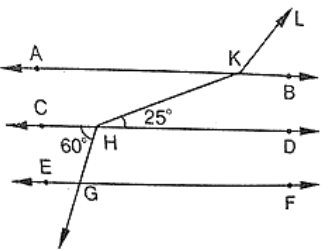Solution:

Produce LK to meet GF at N.

Now, alternative angles are equal

∠CHG = ∠HGN = 60°

∠HGN = ∠KNF = 60°     [Corresponding angles]

Hence, ∠KNG = 180 − 60 = 120

⟹ ∠GNK = ∠AKL = 120° [Corresponding angles]

∠AKH = ∠KHD = 25°     [alternative angles]

Therefore, ∠HKL = ∠AKH + ∠AKL = 25 + 120 = 145°

Question: 4

In the below fig, show that AB ∥ EFSolution:

Produce EF to intersect AC at K.

Now, ∠DCE + ∠CEF = 35 + 145 = 180°

Therefore, EF ∥ CD (Since Sum of Co-interior angles is 180) ... (1)

Now, ∠BAC = ∠ACD = 57°

⟹ BA ∥ EF             [Alternative angles are equal] ... (2)

From (1) and (2)

AB ∥ EF [Since, Lines parallel to the same line are parallel to each other]

Hence proved.

Question: 5

If below fig. if AB ∥ CD and CD ∥ EF, find ∠ACE.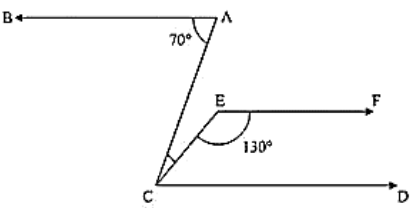Solution:

Since EF ∥ CD

Therefore, EFC + ECD = 180    [co-interior angles are supplementary]

⟹ ECD = 180 - 130 = 50

Also BA ∥ CD

⟹ BAC = ACD = 70          [alternative angles]

But, ACE + ECD = 70

⟹ ACE = 70 - 50 = 20

Question: 6

In the below fig, PQ ∥ AB and PR ∥ BC. If ∠QPR = 102°, determine ∠ABC Give reasons.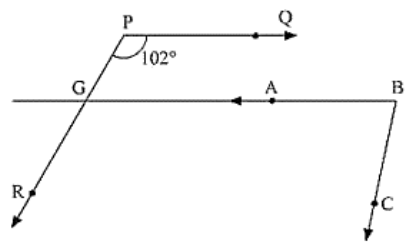Solution:

AB is produce to meet PR at K

Since PQ ∥ AB

∠QPR = ∠BKR = 102°        [corresponding angles]

Since PR ∥ BC

∠RKB + ∠CBK = 180°        [Since Corresponding angles are supplementary]

∠CKB = 180 - 102 = 78

∴  ∠CKB = 78°

Question: 7

In the below fig, state Which lines are parallel and why?Solution:

Vertically opposite angles are equal

⟹ ∠EOC = ∠DOK = 100°

∠DOK = ∠ACO = 100°

Here two lines EK and CA cut by a third line and the corresponding angles to it are equal Therefore, EK ∥ AC.

Question: 8

In the below fig. if l ∥ m, n ∥ p and ∠1 = 85°. find ∠2.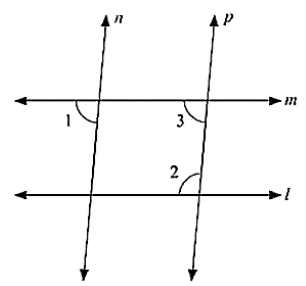Solution:

Corresponding angles are equal

⟹ ∠1 = ∠3 = 85°

By using the property of co-interior angles are supplementary

∠2 + ∠3 = 180°

∠2 + 55 = 180

∠2 = 180 − 85

∠2 = 95°

Question: 9

If two straight lines are perpendicular to the same line, prove that they are parallel to each other.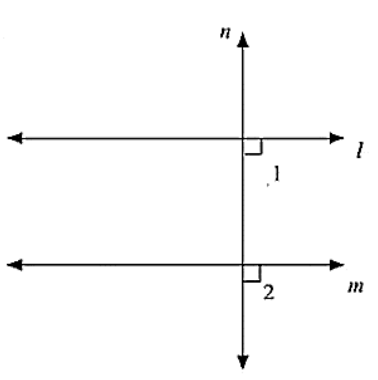Solution:

Given m perpendicular t and l perpendicular to t

∠1 = ∠2 = 90°

Since, I and m are two lines and it is transversal and the corresponding angles are equal

L ∥ M

Hence proved

Question: 10

Prove that if the two arms of an angle are perpendicular to the two arms of another angle. then the angles are either equal or supplementary.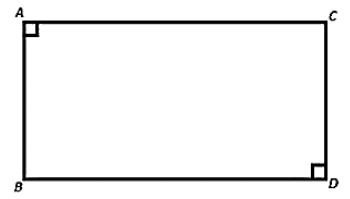Solution:

Consider be angles AOB and ACB

Given 0A perpendicular to A0, also 0B perpendicular to BO

To Prove: ∠AOB + ∠ACB = 180° (or) ∠AOB + ∠ACB = 180°

Proof: In a quadrilateral = ∠A + ∠O + ∠B + ∠C = 360°

[Sum of angles of quadrilateral is 360]

⟹ 180 + O + B + C = 360

⟹ O + C = 360 - 180

Hence AOB + ACB = 180 .... (1)

Also, B + ACB = 180

⟹ ACB = 180 - 90 = ACES = 90°   .... (2)

From (i) and (ii), ACB = A0B = 90

Hence, the angles are equal as well as supplementary.

Question: 11

In the below fig, lines AB and CD are parallel and P is any point as shown in the figure. Show that ∠ABP + ∠CDP = ∠DPB.Solution:

Given that AB ∥ CD

Let EF be the parallel line to AB and CD which passes through P

It can be seen from the figure

Alternative angles are equal

∠ABP = ∠BPF

Alternative angles are equal

∠CDP = ∠DPF

∠ABP + ∠CDP = ∠BPF + ∠DPF

∠ABP + ∠CDP = ∠DPB

Hence proved

AB parallel to CD, P is any point

To prove: ∠ABP + ∠BPD + ∠CDP = 360°

Construction: Draw EF ∥ AB passing through P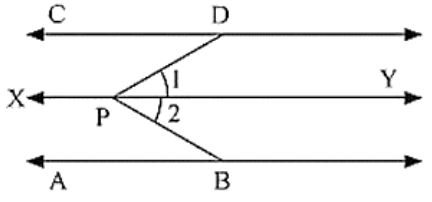Proof:

Since AB ∥ EF and AB ∥ CD, Therefore EF ∥ CD [Lines parallel to the same line are parallel to each other)

∠ABP + ∠EPB = 180° [Sum of co-interior angles is 180)

∠EPD + ∠COP = 180° ....(1) [Sum of co-interior angles is 180)

∠EPD + ∠CDP = 180°  ....(2)

∠ABP + ∠EPB + ∠EPD + ∠CDP = (180 + 180)°

∠ABP + ∠EPB + ∠COP = 360°

Question: 12

In the below fig, AB ∥ CD and P is any point shown in the figure. Prove that: ∠ABP + ∠BPD + ∠CDP = 360°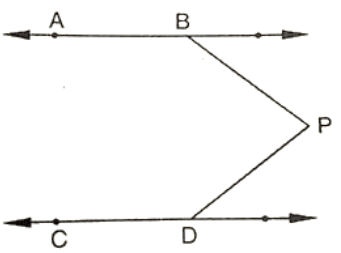Solution:

Through P, draw a line PM parallel to AB or CD.

Now,

A8 || PM

⟹ ABP + BPM = 180

And

CD||PM = MPD + CDP = 180

Adding (i) and (ii), we get A8P + (BPM + MPD) CDP = 360

⟹ ABP + BPD + CDP = 360

Question: 13

Two unequal angles of a parallelogram are in the ratio 2: 3. Find all its angles in degrees.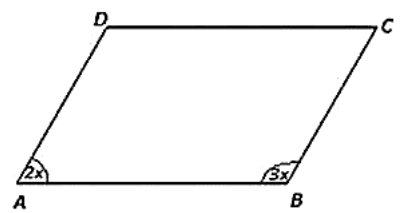Solution:

Let A = 2x and B = 3x

Now, A +B = 180  [Co-interior angles are supplementary]

2x + 3x - 180  [AD II BC and AB is the transversal)

⟹ 5x = 180

x = 180/5

x = 36

Therefore, A = 2 × 36 = 72

b = 3 × 36 = 108

Now, A = C = 72 [Opposite side angles of a parallelogram are equal)

B = D = 108

Question: 14

If each of the two lines is perpendicular to the same line, what kind of lines are they to each other?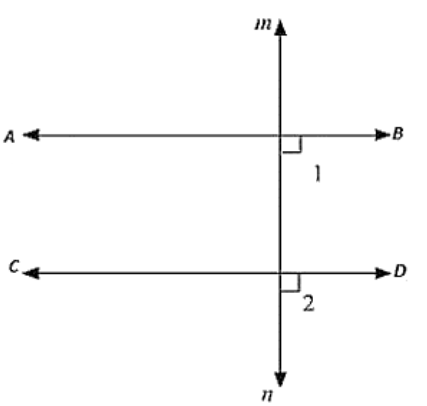Solution:

Let AB and CD be perpendicular to MN

ABD = 90 [AB perpendicular to MN]   ..... (i)

CON = 90 [CO perpendicular to MN]    ....  (ii)

Now, ABD = CDN = 90 (From (i) and (ii))

AB parallel to CD,

Since corresponding angles are equal

Question: 15

In the below fig, ∠1 = 60° and ∠2 = (2/3)rd of a right angle. Prove that l ∥ m.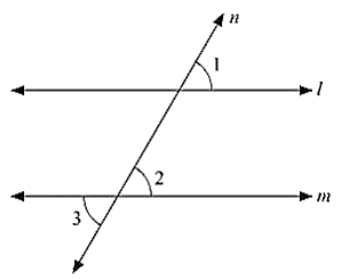Solution:

Given:

∠1 = 60° and ∠2 = (2/3)rd of a right angle

To Prove: Parallel Drawn to m

Proof ∠1 = 60

∠2 = (2/3) × 90 = 60

Since ∠1 = ∠1 = 60°

Therefore, Parallel to m as pair of corresponding angles are equal.

Question: 16

In the below fig, if l ∥ m ∥ n and ∠1 = 60°. Find ∠2.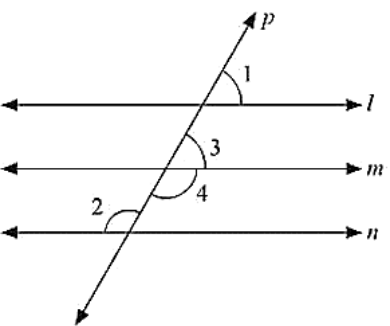Solution:

Since l parallel to m and p is the transversal

Therefore, Given: l ∥ m ∥ n

∠1 = 60°

To find ∠2

∠1 = ∠3 = 60°             [Corresponding angles]

Now, ∠3and∠4 are linear pair of angles

∠3 + ∠4 = 180°

60 + ∠4 = 180

∠4 = 180 - 60

⟹ 120

Also, m ∥ n and P is the transversal

Therefore ∠4 = ∠2 = 120 (Alternative interior angle]

Hence 2∠2 = 120

Question: 17

Prove that the straight lines perpendicular to the same straight line are parallel to one another.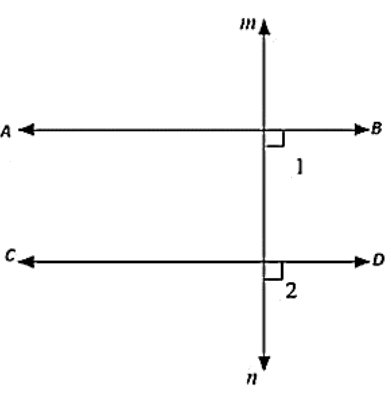Solution:

Let AB and CD be drawn perpendicular to the Line MN

∠ABD = 90°   [AB is perpendicular to MN]  .... (i)

∠CON = 90°   [CD is perpendicular to MN ]  .... (ii)

Now,

∠ABD = ∠CDN = 90° [From (i) and (ii)]

Therefore, AB ∥CD, Since corresponding angles are equal.

Question: 18

The opposite sides of a quadrilateral are parallel. If one angle of the quadrilateral is 60°. Find the other angles.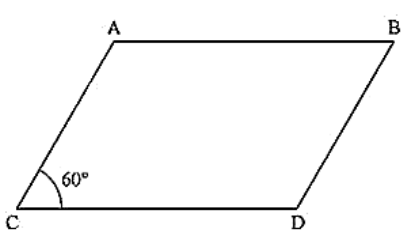Solution:

Given AB ∥ CD

Since AB ∥ CD and AD is the transversal

Therefore, A + D = 180 (Co-interior angles are supplementary)

60 + D = 180

D = 180 - 60

D = 120

Now. AD ∥ BC and AB is the transversal

A + B = 180          (Co-interior angles are supplementary)

60 +B = 180

B = 180 - 60

= 120

Hence, ∠A = ∠C = 60°and∠B = ∠D = 120°

Question: 19

Two lines AB and CD intersect at O. If ∠AOC + ∠COB + ∠BOD = 270°, find the measures of ∠AOC, ∠COB, ∠BOD, ∠DOA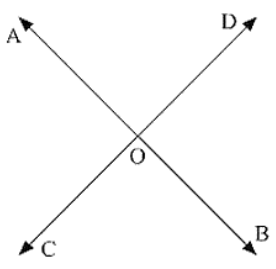Solution:

Given: ∠AOC + ∠COB + ∠BOD = 270°

To find: ∠AOC, ∠COB, ∠BOD, ∠DOA

Here, ∠AOC + ∠COB + ∠BOD = 270° [Complete angle]

⟹ 270 + AOD = 360

⟹ AOD = 360 - 270

⟹ AOD = 90

Now, AOD + BOD = 180         [Linear pair]

90 + BOD = 180

⟹ BOD = 180 - 90

⟹ BOD = 90

AOD = BOC = 90        [Vertically opposite angles]

BOD = AOC = 90        [Vertically opposite angles]

Question: 20

In the below figure, p is a transversal to lines m and n, ∠2 = 120° and ∠5 = 60°. Prove that m|| n.Solution:

Given that

∠2 = 120° and ∠5 = 60°

To prove,

∠2 + ∠1 = 180°   [Linear pair]

120 + ∠1 = 180

∠1 = 180 − 120

∠1 = 60°

Since ∠1 = ∠5 = 60°

Therefore, m ∥ n [As pair of corresponding angles are equal]

Question: 21

In the below fig. transversal t intersects two lines m and n, ∠4 = 110° and ∠7 = 65° is m ∥ n?Solution:

Given:

∠4 = 110° and ∠7 = 65°

To find: is m ∥ n

Here ∠7 = ∠5 = 65°       [Vertically opposite angle]

Now. ∠4 + ∠5 = 110 + 65 = 175°

Therefore, m is not parallel to n as the pair of co interior angles is not supplementary.

Question: 22

Which pair of lines in the below fig. is parallel? Give reasons.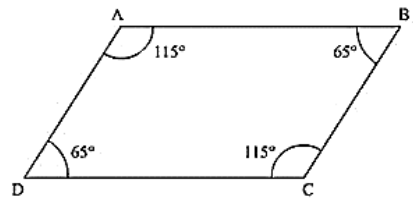Solution:

∠A + ∠B = 115 + 65 = 180°

Therefore, AB ∥ BC [As sum of co interior angles are supplementary]

∠B + ∠C = 65 + 115 = 180°

Therefore, AB ∥ CD (As sum of interior angles are supplementary]

Question: 23

If I, m, n are three lines such that I∥ m and n perpendicular to l, prove that n perpendicular to m.Solution:

Given, l ∥ m, n perpendicular to I

To prove: n perpendicular to m

Since l ∥ m and n intersects

∴ ∠1 = ∠2             [Corresponding angles]

But, U = 90

⟹ ∠2 = 90°

Hence n is perpendicular to m

Question: 24

In the below fig, arms BA and BC of ∠ABC are respectively parallel to arms ED and EF of ∠DEF. Prove that ∠ABC = ∠DEF.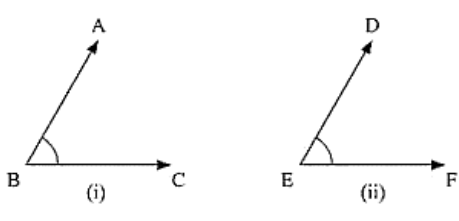Solution:

Given

AB ∥ DE and BC ∥ EF

To Prove: ∠ABC = ∠DEF

Construction: Produce BC to x such that it intersects DE at M.

Proof: Since AB ∥ DE and BX is the transversal

ABC = DMX                             [Corresponding angle]  .... (i)

Also, BX ∥ EF and DE is the transversal

DMX = DEF                             [Corresponding angles]         -----(ii)

From (i) and (ii)

∠ABC = ∠DEF

Question: 25

In the below fig, arms BA and BC of ABC are respectively parallel to arms ED and EF of DEF Prove that ∠ABC + ∠DEP = 180°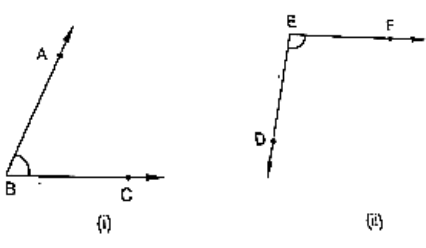Solution:

Given:

AB ∥ DE, BC ∥ EF

To prove: ∠ABC + ∠DEF = 180°

Construction: Produce BC to intersect DE at M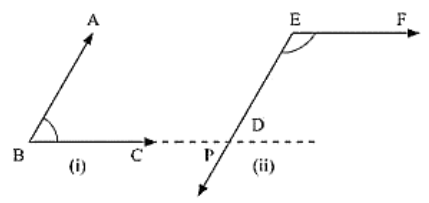Proof:

Since AB || EM and BL is the transversal

∠ABC = ∠EML         [Corresponding angle] ... (i)

Also,

EF || ML and EM is the transversal

By the property of co-interior angles are supplementary

∠DEF + ∠EML = 180°  ....  (ii)

From (i) and (ii) we have

Therefore ∠DEF + ∠ABC = 180°

Question: 26

With of the following statements are true (T) and which are false (F)? Give reasons.

(1) If two lines are intersected by a transversal, then corresponding angles are equal.

(ii) If two parallel lines are intersected by a transversal, then alternate interior angles are equal.

(ii) Two lines perpendicular to the same line are perpendicular to each other.

(iv) Two lines parallel to the same line are parallel to each other.

(v) If two parallel lines are intersected by a transversal, then the interior angles on the same side of the transversal are equal.

Solution:

(i) False

(ii)True

(iii) False

(iv) True

(v) False

Question: 27

Fill in the blanks in each of the following to make the statement true:

(i) If two parallel lines are intersected by a transversal, then each pair of corresponding angles are ____________

(ii) If two parallel lines are intersected by a transversal, then interior angles on the same side of the transversal are _____________

(iii) Two lines perpendicular to the same line are _______ to each other

(Iv) Two lines parallel to the same line are __________ to each other.

(v) If a transversal intersects a pair of lines in such a way that a pair of alternate angles we equal. then the lines are ___________

(vi) If a transversal intersects a pair of lines in such a way that the sum of interior angles on the seine side of transversal is 180'. then the lines are _____________

Solution:

(i) Equal

(ii) Parallel

(iii) Supplementary

(iv) Parallel

(v) Parallel

(vi) Parallel
```### Course Features

• 728 Video Lectures
• Revision Notes
• Previous Year Papers
• Mind Map
• Study Planner
• NCERT Solutions
• Discussion Forum
• Test paper with Video Solution